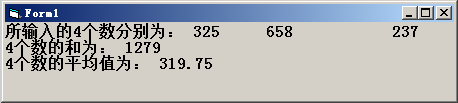﻿ VB编程：从键盘上输入4个数，编写程序，计算并输出这4个数的和及平均值。通过inputbox函数输入数据，在窗体上显示和及平均值。 - VB程序设计教程答案

# VB编程：从键盘上输入4个数，编写程序，计算并输出这4个数的和及平均值。通过inputbox函数输入数据，在窗体上显示和及平均值。

###### 2016-06-30 15:12:38  分类: VB程序设计教程第4版答案刘炳文  参与: AddViewCount(1)人

Sub Form_Click()
a = InputBox(w输入第一个数"> a — Val(a)
b = InputBox(w输人第二个数") b = Val(b)
c = InputBox("输人第三个数 c = VaKc)
d = InputBox(w输人第四个数"） d = Val(d)
sum = a + b+ c + d aver = sum / 4Private Sub Form_Click()
Dim a As Single
For i = 1 To 4
a = InputBox("请输入一个数：", "数据输入")
Sum = Sum + a
n = n + 1
Avg = Sum / n
Next i
Print "和是：", Sum
Print "平均值是：", Avg

End Sub